# Positive derivative implies increasing

(diff) ← Older revision | Latest revision (diff) | Newer revision → (diff)

## Statement

### On an open interval

Suppose$f$ is a function on an open interval$I$ that may be infinite in one or both directions (i..e,$I$ is of the form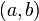$\! (a,b)$,$(a,\infty)$,$(-\infty,b)$, or$(-\infty,\infty)$). Suppose the derivative of$f$ exists and is positive everywhere on$I$, i.e.,$\! f'(x) > 0$ for all$x \in I$. Then,$f$ is an increasing function on$I$, i.e.: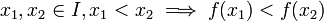$x_1,x_2 \in I, x_1 < x_2 \implies f(x_1) < f(x_2)$

### On a general interval

Suppose$f$ is a function on an interval$I$ that may be infinite in one or both directions and may be open or closed at either end. Suppose$f$ is a continuous function on all of$I$ and that the derivative of$f$ exists and is positive everywhere on the interior of$I$, i.e.,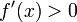$f'(x) > 0$ for all$x \in I$ other than the endpoints of$I$ (if they exist). Then,$f$ is an increasing function on$I$, i.e.:$x_1,x_2 \in I, x_1 < x_2 \implies f(x_1) < f(x_2)$

## Proof

### General version

Given: A function$f$ on interval$I$ such that$f'(x) > 0$ for all$x$ in the interior of$I$ and$f$ is continuous on$I$. Numbers$x_1 < x_2$ with$x_1, x_2 \in I$.

To prove:$\! f(x_1) < f(x_2)$

Proof:

Step no. Assertion/construction Facts used Given data used Previous steps used Explanation
1 Consider the difference quotient$\! \frac{f(x_2) - f(x_1)}{x_2 - x_1}$. There exists$x_3$ such that$x_1 < x_3 < x_2$ and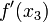$\! f'(x_3)$ equals this difference quotient. Fact (1)$x_1 < x_2$,$f$ is defined and continuous on an interval$I$ containing$x_1,x_2$, differentiable on the interior of the interval. [SHOW MORE]
2 The difference quotient$\! \frac{f(x_2) - f(x_1)}{x_2 - x_1}$ is positive.$f'(x)$ is positive for all$x$ in the interior of$I$. Step (1) [SHOW MORE]
3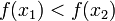$f(x_1) < f(x_2)$$x_1 < x_2$ Step (2) [SHOW MORE]